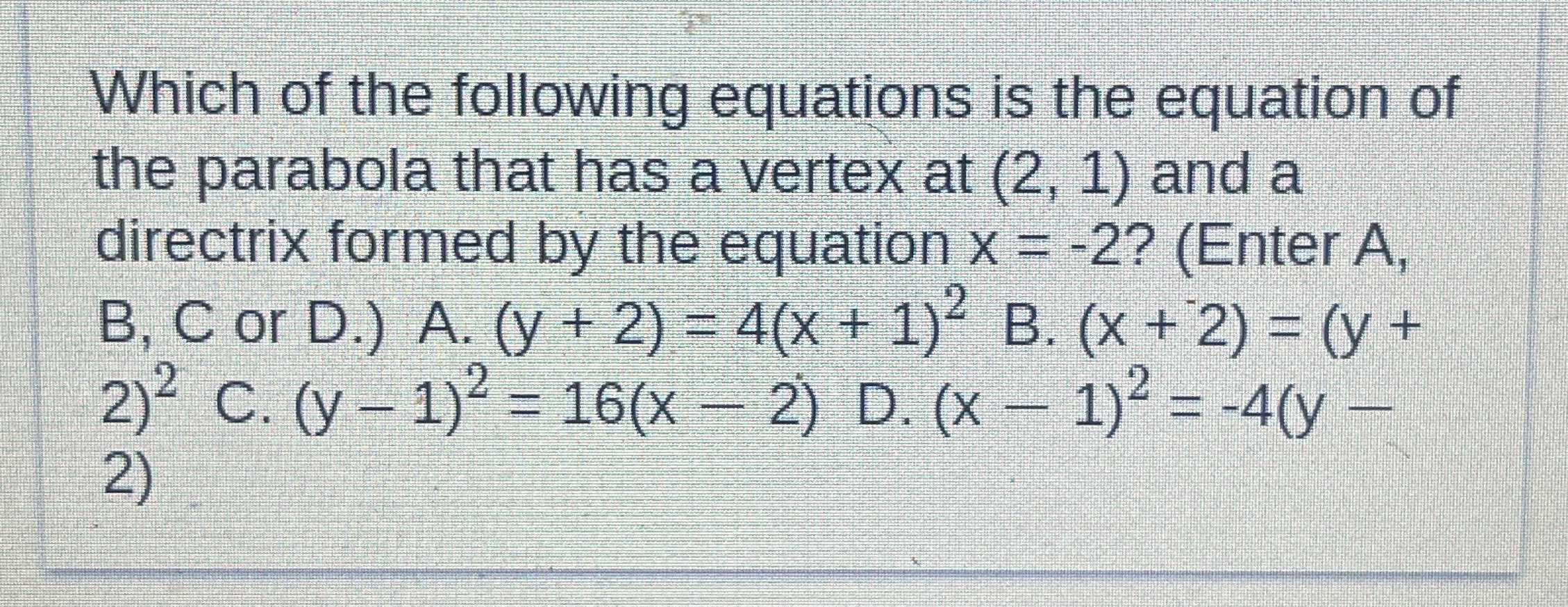### Still have math questions?

Algebra
QuestionWhich of the following equations is the equation of the parabola that has a vertex at $$( 2,1 )$$ and a directrix formed by the equation $$x = - 2 ?$$ (Enter $$A$$ , B, C or $$D . )$$

A.$$( y + 2 ) = 4 ( x + 1 ) ^ { 2 }$$

B. $$( x + 2 ) = ( y + 2)^2$$

C. $$( y - 1 ) ^ { 2 } = 16 ( x - 2 )$$

D. $$( x - 1 ) ^ { 2 } = - 4 ( y - 2)$$